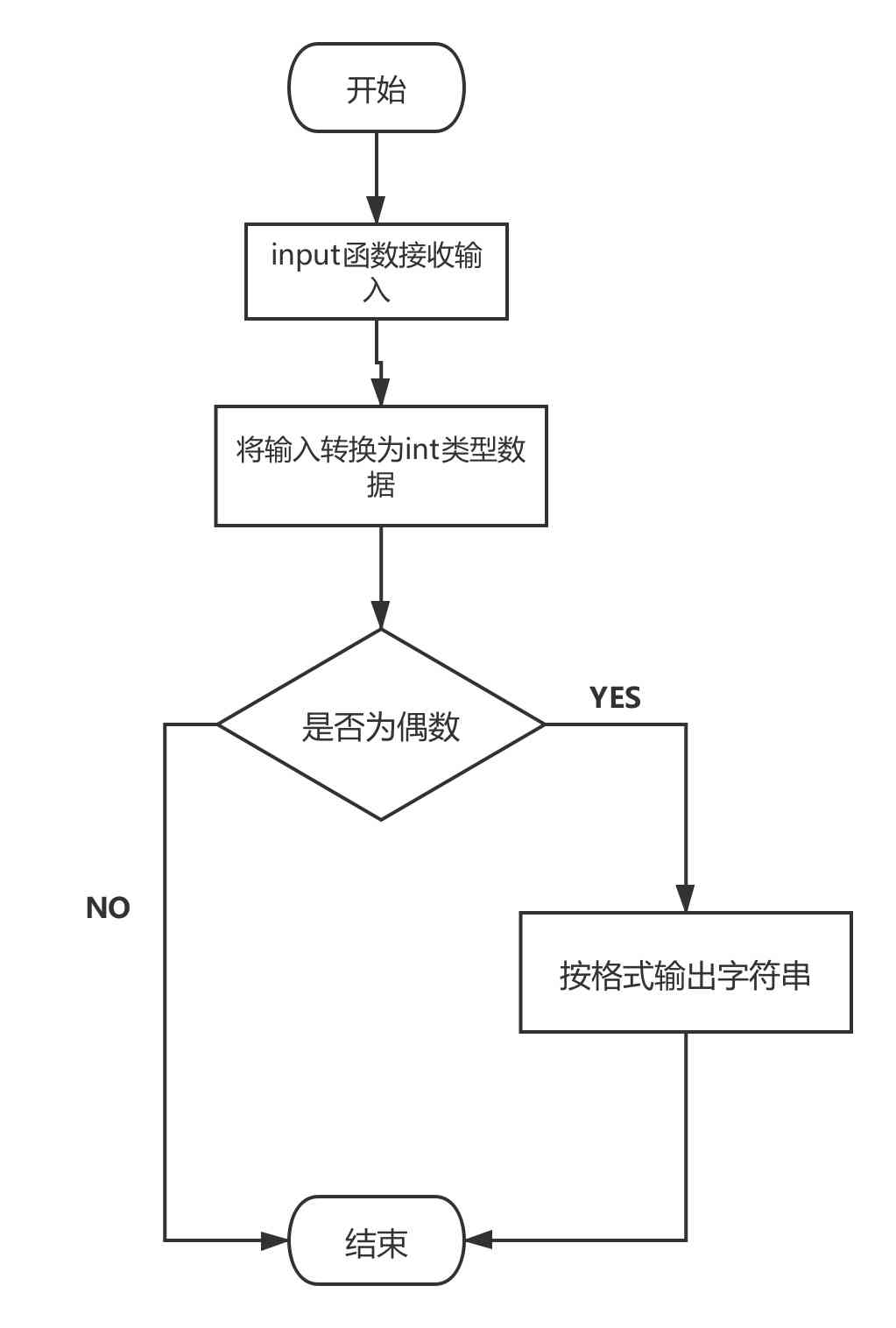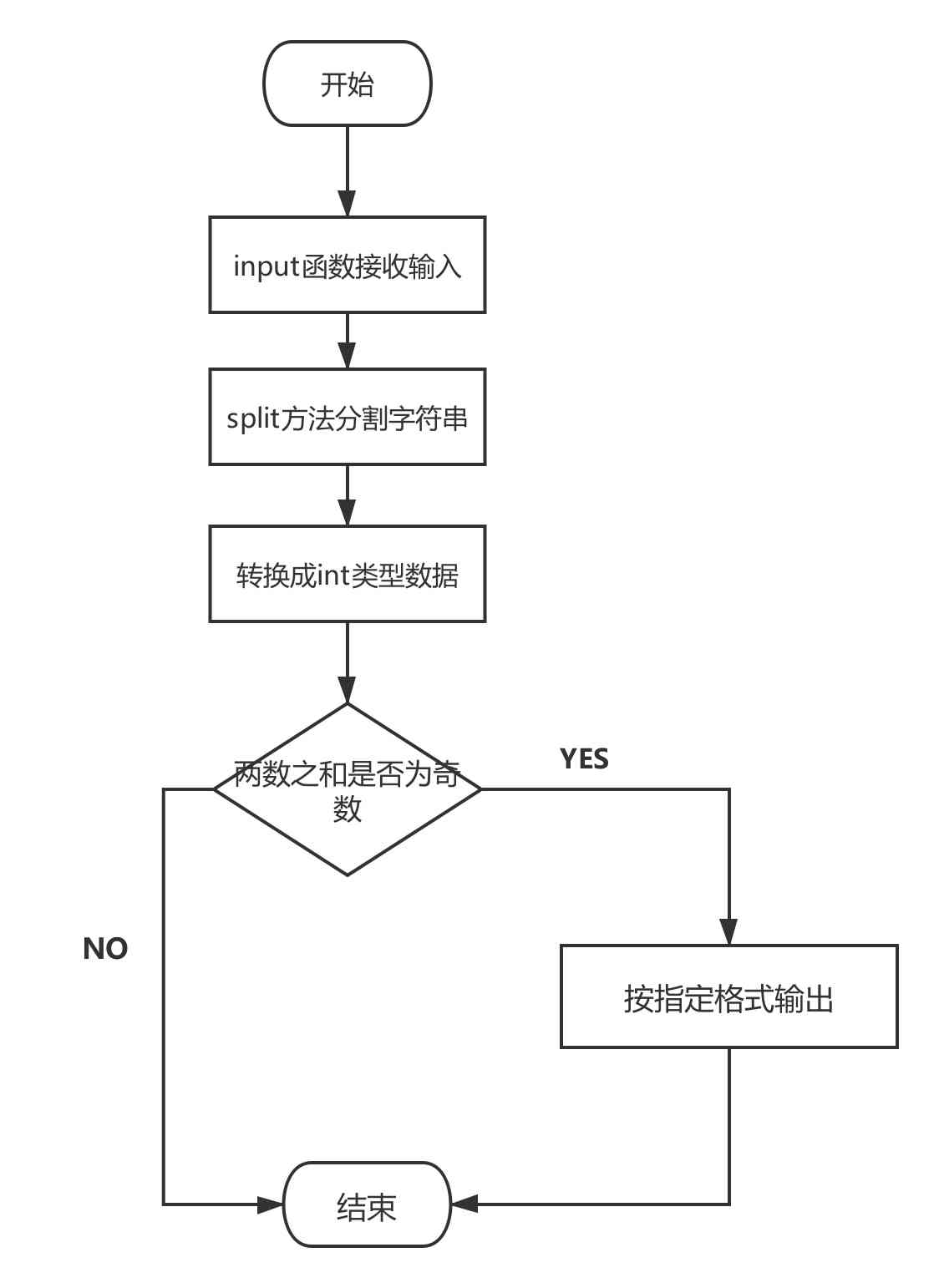# python 条件控制 if语句

python编程中 if语句用于控制程序的执行， if分支使用布尔表达式或布尔值作为分支条件来进行分支控制， if关键字后面的表达式如果为真,则进入到if语句块执行代码， 程序通过if语句中对条件表达式的判断进入到不同的逻辑分支中。

## 1. if 语句

``````if condition:
block
``````
• condition 是一个表达式
• 如果condition等价于True,则进入block代码块执行代码
• block代码块相比于if语句缩进4个空格

``````value = input("请输入一个整整数:")
i_value = int(value)
if i_value % 2 == 0:
print("你输入的整数是:{value}, 它是偶数".format(value=value))
``````
1. input接收用户输入，返回的是字符串
2. 将用户输入数据转成int类型数据
3. 使用if语句，如果数据是偶数，则输出它是偶数的结论

1. input函数
2. int函数
3. if条件语句
4. % 取模运算
5. 字符串格式化## 2. 对程序要有绝对掌握权

### 2.2 示例代码

``````value = input("输入两个正整数,中间用逗号隔开:")
value_lst = value.split(",")
value_1, value_2 = int(value_lst), int(value_lst)
if (value_1 + value_2) % 2 == 1:
print("{var1} 和 {var2} 的和是奇数".format(var1=value_1, var2=value_2))
``````

### 2.3 程序分析，绝对的掌握权

1. input函数返回的数据是字符串
2. 使用字符串的split方法，可以用分割符对字符串进行分割。split方法返回的是列表，那么value_lst就是一个列表，它的值是 ['12', '13']
3. 两个字符串不能进行数字的加法操作，因此将他们转换成int类型数据，并赋值给两个新变量
4. value_1与value_2的和是25，对2取模的结果是1，表达式 1==1 的结果是True,if 条件成立
5. 按照指定格式输出

### 2.4 程序流程图QQ交流群: 211426309